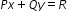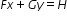# Sample Test Item PreviewExport Print
General Information
Related Benchmark: MAFS.912.A-REI.3.5
Reporting Category: Algebra: Reasoning with Equations & Inequalities
Type: MS: Multiselect
Difficulty: N/A
Question:

The systemhas the solution (3,-1) where F,G,H,P,Q and R are nonzero real numbers.Select all the systems that are also guaranteed to have the solution (3,-1).

A.

(P+F)x +(Q+G)y=R+H

Fx+Gy=H

B.

(P+F)x+Qy=R+H
Fx+(G+Q)y=h

C.

Px+Qy=R

(3P+F)x+(3Q+G)y=3H+R

D.

Px+Qy=R

(F-2P)x+(G-2Q)y=H-2R

E.

Px+Qy=R

5Fx+5Gy=5H